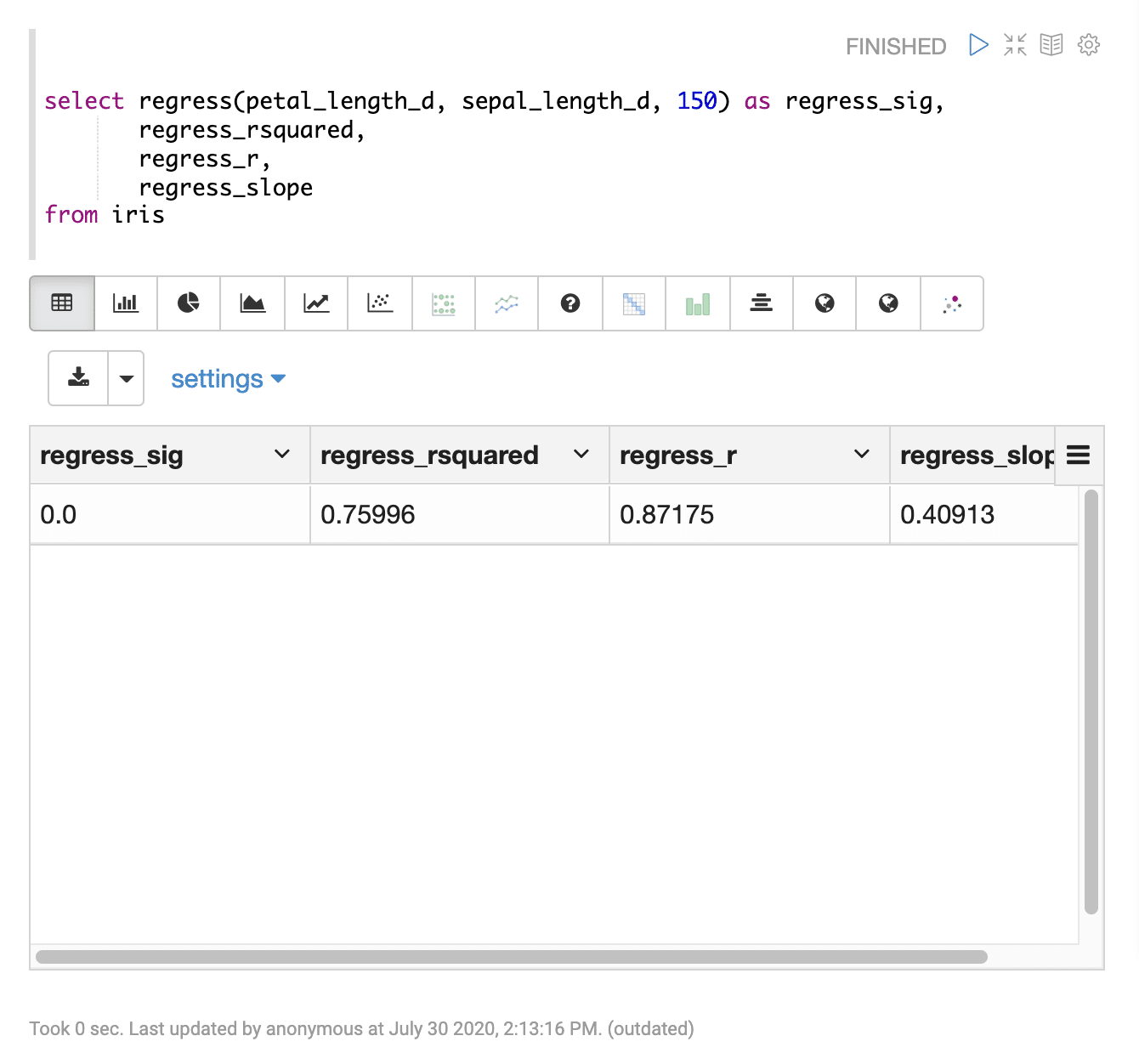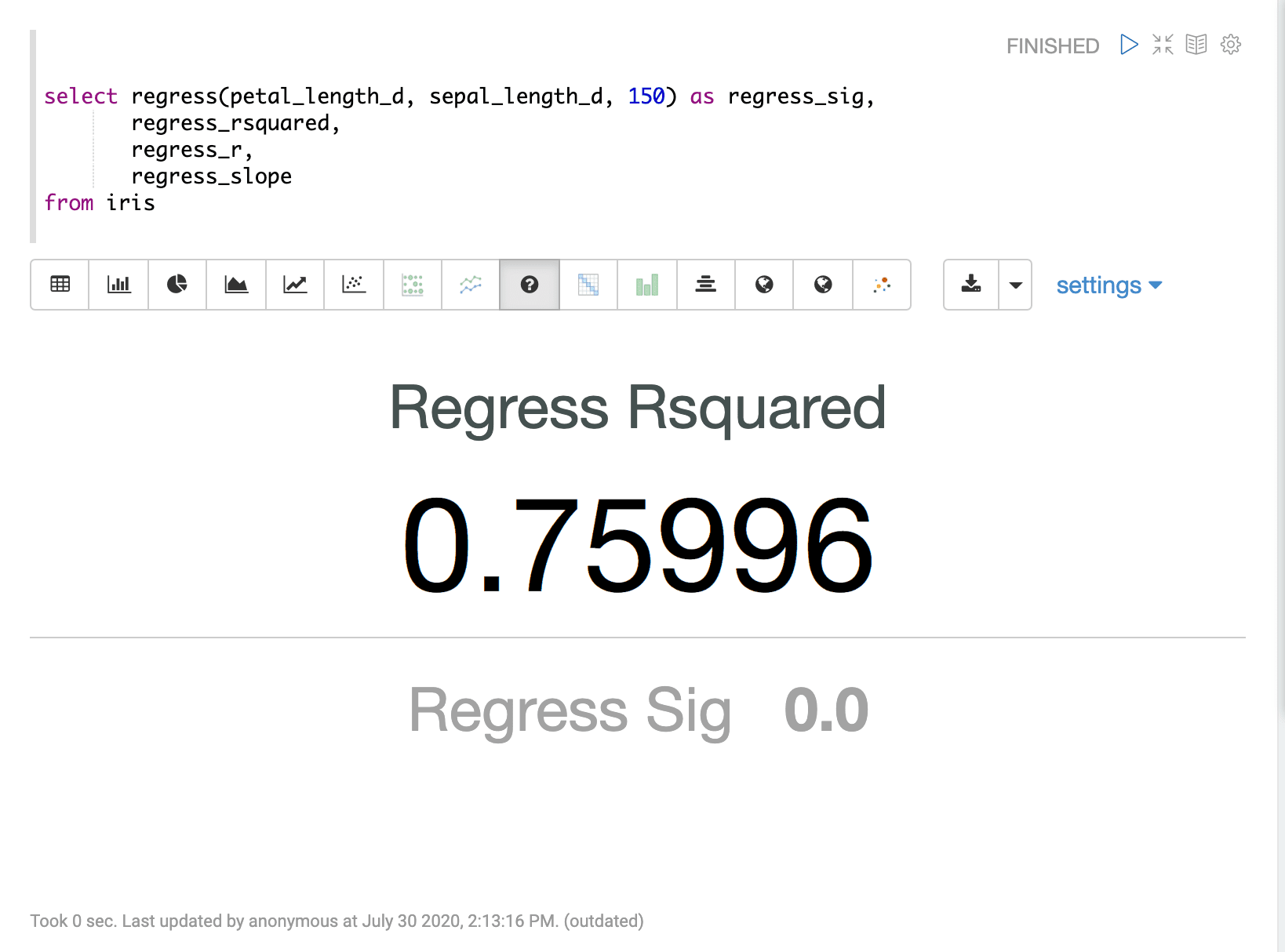# Regression Diagnostics (regress)

The `regress` function computes diagnostics for bi-variate linear regression. The `regress` function takes three parameters:

1. The numeric field of the independent variable (x).

2. The numeric field of the dependent variable (y).

3. The sample size of the regression.

## Sample syntax

``````select regress(petal_length_d, sepal_length_d, 150) as regress_sig,
regress_rsquared,
regress_r,
regress_slope
from iris``````

## Result set

The result set for the `regress` function has one record that contains the selected regression diagnostics. The `regress` function returns the statistical significance of the regression analysis. The following regression diagnostics can be selected as well:

• `regress_slope` (slope)

• `regress_intercept` (y-intercept)

• `regress_rsquared` (R Squared)

• `regress_r` (correlation coefficient)

• `regress_mse` (mean square error)

• `regess_sse` (sum square error)

• `regress_ssr` (sum square due to regression)

• `regress_ssto` (total sum of squares)

Sample `regress` result in Apache Zeppelin## Visualization

Sample visualization of the `regress` function using Apache Zeppelin’s Number visualization.